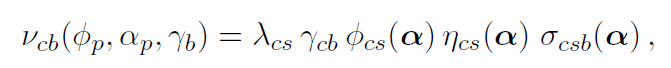# How can I get expected number of events from HistFactory model?

Dear expert,
I want to ask about the way to get the expected number of events from HistFactory.

According to HistFactory document,is the expected number of events for each channel and each bin. I want to get this \nu_{cb} from workspace which is made by HistFactory.

Is there any function like

TFile* f = TFile::Open("workspace.root");
RooWorkspace* w = (RooWorkspace*)f->Get("combined");
ModelConfig* mc = (ModelConfig*)w->obj("ModelConfig");

double expected_evt_channel0_bin1 = w->function("nu_expected_evts_ch0_bin1")->getVal(); // this is what I want!


PS.
I want to get this value for Toy MC study. Because I want to check fit quality, I produce 5k Toys and do a fitting for each of Toy samples:

double Nevt_total = ??? // expected number of events... It is what I want to know

ModelConfig* mc = (ModelConfig*)w->obj("ModelConfig");
RooSimultaneous* model = (RooSimultaneous*)mc->GetPdf();
RooDataSet* genData = model->generate(RooArgSet(*x,model->indexCat()), Nevt_total, false, false, "", false, true);
RooFitResult* fitres = model->fitTo(*genData, RooFit::SumW2Error(false), PrintLevel(-1), Save());


When I produce Toy samples, I change systematic uncertainties like HistoSys and OverallSys. If these systematics change, the expected number of events for each bin also changes. Therefore, Nevt_total in the above code should be changed.

Thank you

@moneta or @jonas can you help here? Thanks!

Hi @june0812,

Here is a function I wrote that you can use to get the expected number of events from a HistFactory model:

// Compute the expected number of events for a binned model created by
// HistFactory.
double expectedEventsFromHistFactoryModel(RooWorkspace const &ws,
std::string const& simPdfName="simPdf")
{
// magic constants to get the names right
std::string const obsPrefix = "obs_x_channel";
std::string const channelPrefix = "channel";
std::string const channelSuffix = "_model";

// get the top-level pdf
auto *model = static_cast<RooSimultaneous *>(ws.pdf(simPdfName.c_str()));

// empty normalization set to get raw yields
RooArgSet emptySet{};

double expectedEvents = 0.0;

// loop over channels
for (std::size_t iChannel = 0; iChannel < model->indexCat().size(); ++iChannel) {

// temporary to build the observable and pdf names
auto const iChanStr = std::to_string(iChannel + 1);

// get the observable in that channel and its binning
RooRealVar &obs = *ws.var((obsPrefix + iChanStr).c_str());
RooAbsBinning const &binning = obs.getBinning();

// get the RooRealSumPdf that represents the yields divided by the bin width
RooAbsPdf const &channelPdf = *ws.pdf((channelPrefix + iChanStr + channelSuffix).c_str());

// remember the original value to reset later
const double oldVal = obs.getVal();

// loop over bins
for (std::size_t iBin = 0; iBin < binning.numBins(); ++iBin) {
double binCenter = binning.binCenter(iBin);
double binWidth = binning.binWidth(iBin);

// set the observable to the bin center before evaluating the channel
// pdf for that observable value, and multiply with bin width to get
// the real yields
obs.setVal(binCenter);
expectedEvents += channelPdf.getVal(emptySet) * binWidth;
}

obs.setVal(oldVal); // just to make sure we don't change the state
}

return expectedEvents;
}


I made a few comments for you to follow along with what it does. Essentially you just need to iterate in a double-loop over channels and bins, using the right objects in the workspace to get the bin yields. If you do w->Print() on your workspace you should be able to find these observable and channel PDF objects that the function is using. Apparently you renamed the simultaneous PDF to “combined”, so the input parameter simPdfName needs to be set accordingly.

Let me know if this works!

Cheers,
Jonas

@jonas, Thank you! It works fine.

However, I found that your solution only works when the sizes of bins are the same. Even though the total number of event is the same, your code shows different value when I change the sizes of bins.

Alternatively, I found a way to count the expected number of event. In the workspace, there are RooProduct like L_x_Signal_nominal_channel_overallSyst_x_StatUncert, where Signal_nominal is the name of my histogram. This function has the expected number of events. Therefore, following code is possible:

RooWorkspace *w;
RooRealVar* obs = w->var("obs_x_channel");
*obs = 0.5;
RooAbsReal * function = w->function("L_x_Signal_nominal_channel_overallSyst_x_StatUncert");
expectedEvents = function->getValV(); // expected number of event at obs = 0.5


This topic was automatically closed 14 days after the last reply. New replies are no longer allowed.